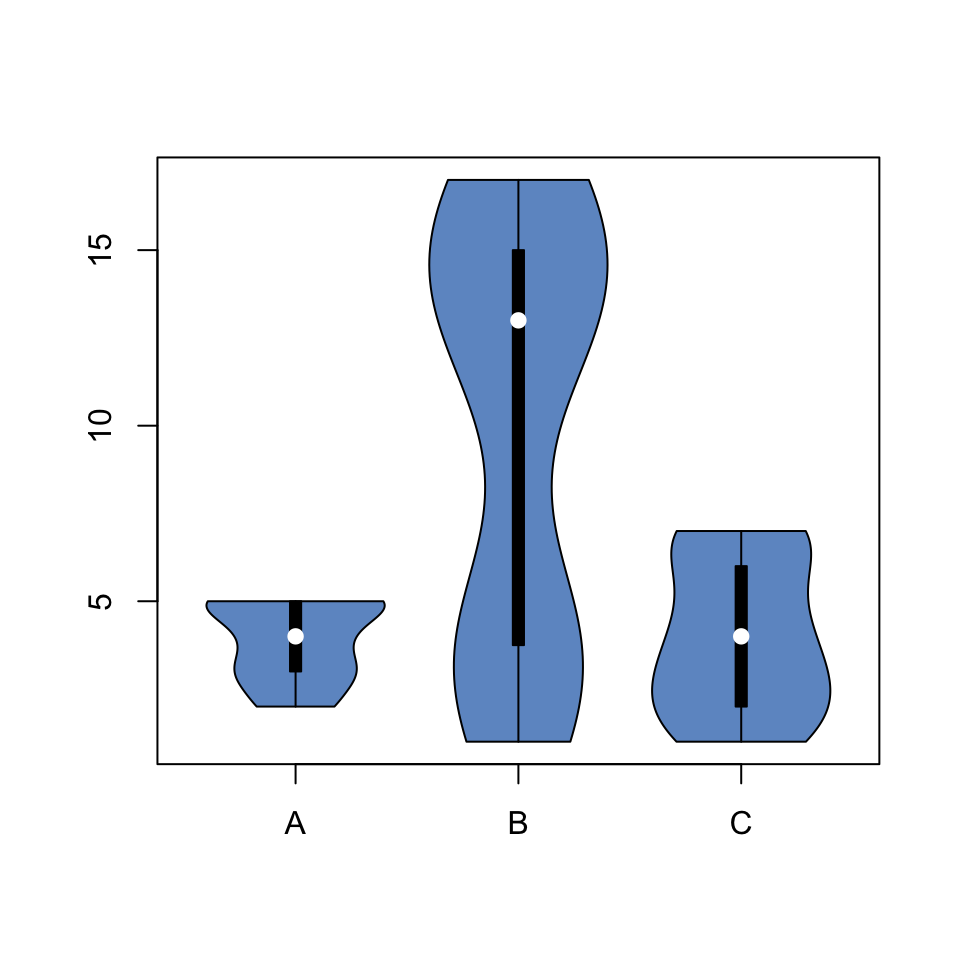# Violin Plot with the vioplot package

Violin plots are useful to compare the distribution of several groups. I strongly advise to use ggplot2 to build them, but the `vioplot` library is an alternative in case you don’t want to use the `tidyverse`.

The `Vioplot` library builds the violin plot as a boxplot with a rotated kernel density plot on each side. If you want to represent several groups, the trick is to use the `with` function as demonstrated below.

Note: consider using the ggplot2 package as shown in graph #95.

``##   1 17````````# Load the vioplot library
library(vioplot)

# Create data
treatment <- c(rep("A", 40) , rep("B", 40) , rep("C", 40) )
value <- c( sample(2:5, 40 , replace=T) , sample(c(1:5,12:17), 40 , replace=T), sample(1:7, 40 , replace=T) )
data <- data.frame(treatment,value)

# Draw the plot
with(data , vioplot(
value[treatment=="A"] , value[treatment=="B"], value[treatment=="C"],
col=rgb(0.1,0.4,0.7,0.7) , names=c("A","B","C")
))``````

Related chart types

## Contact

This document is a work by Yan Holtz. Any feedback is highly encouraged. You can fill an issue on Github, drop me a message on Twitter, or send an email pasting yan.holtz.data with gmail.com.